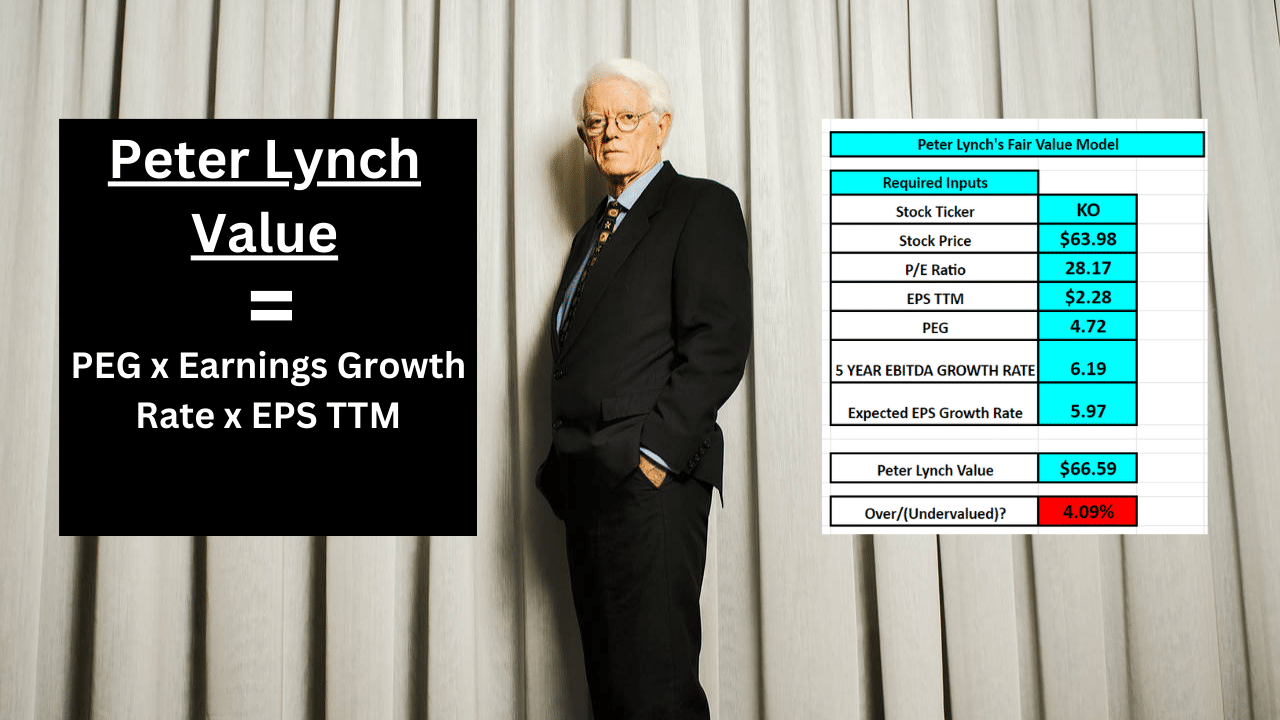# Is the Gordon Growth Model Accurate in Working Out the Intrinsic Value of a Stock

• By: Walter Dunphy ACCA
• Date: May 26, 2023
• Time to read: 4 min.

As the saying goes, there is more than one way to skin a cat. Well, there is also more than one way to try and work out the intrinsic value of a stock. In this blog post, we will be taking a close look at one method in particular – the Gordon Growth Valuation Model, also known as the Dividend Discount Model (DDM).

### Understanding the Formula

D1 = is the dividend expected to be received at the end of the first year.

Typically we like to use Dividend.com as our primary resource for calculating D1. You can take the company’s most recent dividend and then multiply that by the expected growth rate over the next year to work out D1.

r = is the required rate of return or discount rate

The most commonly used proxy for the required rate of return in the Gordon Growth Model is the company’s cost of equity. We already have created a WACC (weighted average cost of capital) calculator, of which one if its component parts is calculating the cost of equity, make sure to check it out.

g = is the expected constant growth rate of dividends

As mentioned already, Dividend.com is a good resource to go to for estimated future dividend growth rates. Dividend.com reference the expected average annual growth rate over the next 1Y, 3Y, 5Y, 10Y, and 20Y. Here you will need to use your own judgement of what the appropriate growth rate to use is.

The Gordon Growth Model is a popular method in calculating the intrinsic value of a stock as it is a relatively simply calculation to compute with just a few inputs.

The model assumes that dividends will grow at a constant rate into the future and based on this we can estimate a stock’s intrinsic value by discounting future dividends back by the cost of equity less the dividend growth rate – which gives you the present value of

For example, we tested it out using the Coca-Cola Company (KO) as our test subject.

When all variables are input, the output of the calculation was that Coca-Cola is slightly overvalued by 2.4%. Based on this example, it looks like Gordon’s Growth Model may have some potential in accurately valuing stocks, but as we will discuss now, there are also many weaknesses associated with this model.

### What are the weaknesses of the Gordon Growth Model for valuing stocks?

The Gordon Growth Valuation model is only as good as the assumptions fed into the formula. The two key variables r and g can be estimated differently by preparers based. As a result of this two people could use Gordon’s Growth Model to calculate the intrinsic value of the sale company and come up with different results.

Not only that, this formula is particularly sensitive to small changes in the assumptions made. If i take the example from above and change the dividend growth rate for KO and change it to 5%, it goes from being 2.3% overvalued to 12% undervalued. Therefore, when you are working with the Gordon Growth Model it is wise to add in a margin of safety of 20-30% to ensure you don’t make any fatal errors.

Another vital assumption this model makes is that dividends will grow at a constant stable rate. In reality, due to various reasons a company may have to stop paying dividends, or pauses dividend increases during tough economic conditions. This means the Gordon Growth Model may not be suitable for all dividend paying stocks.

A metric worth checking out (also available on Dividend.com) is how many years has the company consecutively increased dividends. For example, The Coca-Cola Company (KO) has had 62 years of dividend increases.

Ignoring other important valuation factors is another weakness of the Gordon Growth model, which only focuses solely on dividends and does not consider other factors that can influence a stock’s value, such as earnings, cash flows, or market conditions. It assumes that dividends are the sole driver of value for investors, overlooking the potential impact of factors like changes in interest rates, competitive dynamics, or overall market sentiment.

If you are interested in learning about some other valuation models then please check out the Peter Lynch’s valuation model and the Discounted Cash Flow model.

Disclaimer: This blog post is for informational and educational purposes only and should not be construed as financial advice.Previous Post